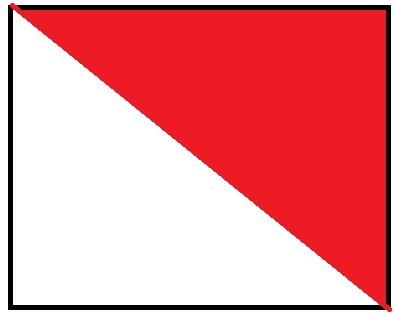# 11.盛最多水的容器

## 一、题目描述## 二、解题方案

### 一、暴力法int maxArea(vector<int>& height) {
if (height.size() == 0) return 0;

int max= 0,temp =0;
for (int i = 0; i < height.size(); i++) {
for (int j = i+1; j < height.size(); j++) {
int temp = (j - i)*(height[i] < height[j] ? height[i] : height[j]);
max= max> temp ? max : temp;
}
}
return max;
}


### 双指针法

int maxArea(vector<int>& height) {
if (height.size() == 0) return 0;

int begin = 0,end ,max = 0;
end = height.size()-1;

while (begin != end) {
int w= end - begin;
int h = height[begin] > height[end] ? height[end] : height[begin];
max = max > w*h ? max : w * h;
if (height[begin] > height[end]) end--;
else begin++;
}
}

03-2598704-12313
01-27468
09-031515
06-09132
08-081130
05-0695
03-26434
04-28106
01-17132